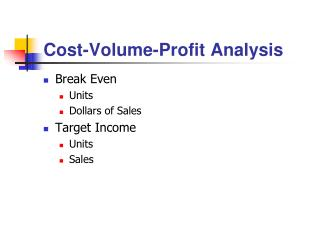# Cost-Volume-Profit Analysis - PowerPoint PPT PresentationDownload PresentationCost-Volume-Profit Analysis

Cost-Volume-Profit AnalysisDownload Presentation## Cost-Volume-Profit Analysis

- - - - - - - - - - - - - - - - - - - - - - - - - - - E N D - - - - - - - - - - - - - - - - - - - - - - - - - - -
##### Presentation Transcript

1. Cost-Volume-Profit Analysis • Break Even • Units • Dollars of Sales • Target Income • Units • Sales

2. Cost-Volume-Profit Analysis • Multiple Products • Assume constant sales mix • CMU for each product • Then calculate break even based on sales mix • Apples CMU \$1; Oranges CMU \$.50 • Fixed costs: \$100 • Sales mix: 40% apples, 60% oranges • Breakeven units?

3. Cost-Volume-Profit Analysis • Evaluating alternatives • Increase in CM – Increase in Fixed Costs • Margin of safety • Sales – breakeven sales • Can be in units or dollars • Assume constant sales mix

4. Cost-Volume-Profit Analysis • Operating leverage = CM/Operating Income • Cost structure: variable versus fixed costs • Higher fixed costs = more risk • Higher fixed costs better = lower variable costs • Higher fixed costs better = higher CMU • Higher CMU = greater increase in income for each increase in units sold